# Using Symmetry in DesignModeler and Expanding the Results

If we want to have a half model of this…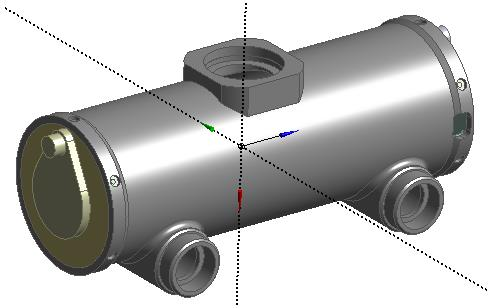In DM, click Tools, Symmetry then select the Plane. Usually the geometry is a Full Model.

(Sometimes only one side of the part has been created before a mirror feature completes the part. In this case, then the Model Type is Partial Model.)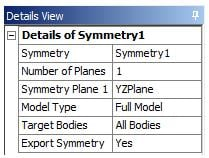Generate and this is the result.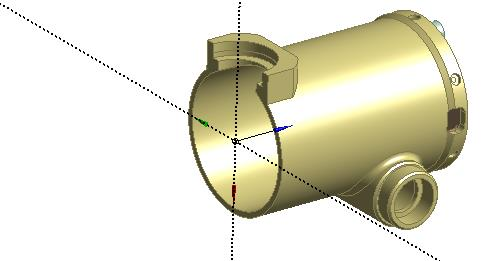If you want the other half, you have to create a plane where the Z axis points to the half you want to keep. In this case I created Plane4.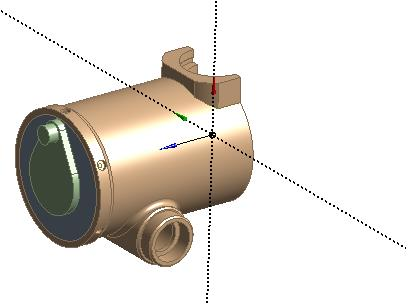In Mechanical, build a model and get a solution.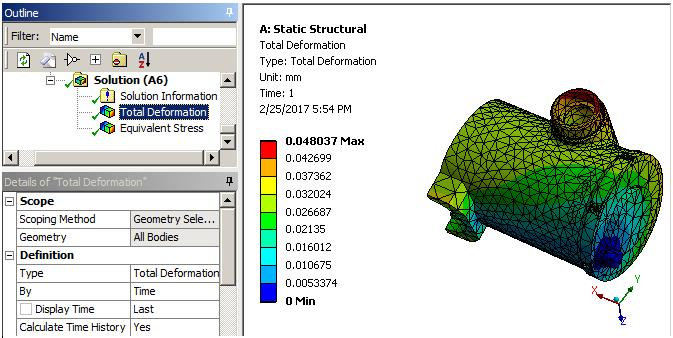In Workbench, Tools, Options, Appearance, turn on Beta Options (one time configuration).

In Mechanical, click on the Symmetry item to get the details window.

Set Num Repeat to 2 and Method to Half and put 1e-3 into ?Z.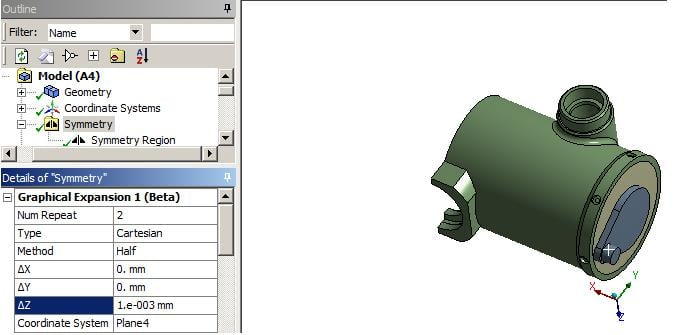Click on the Total Deformation and you will have the symmetry expanded.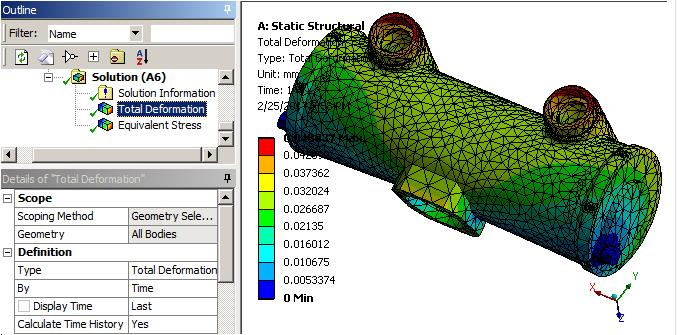«1

• Here is a fluid flow example: A quarter symmetry model.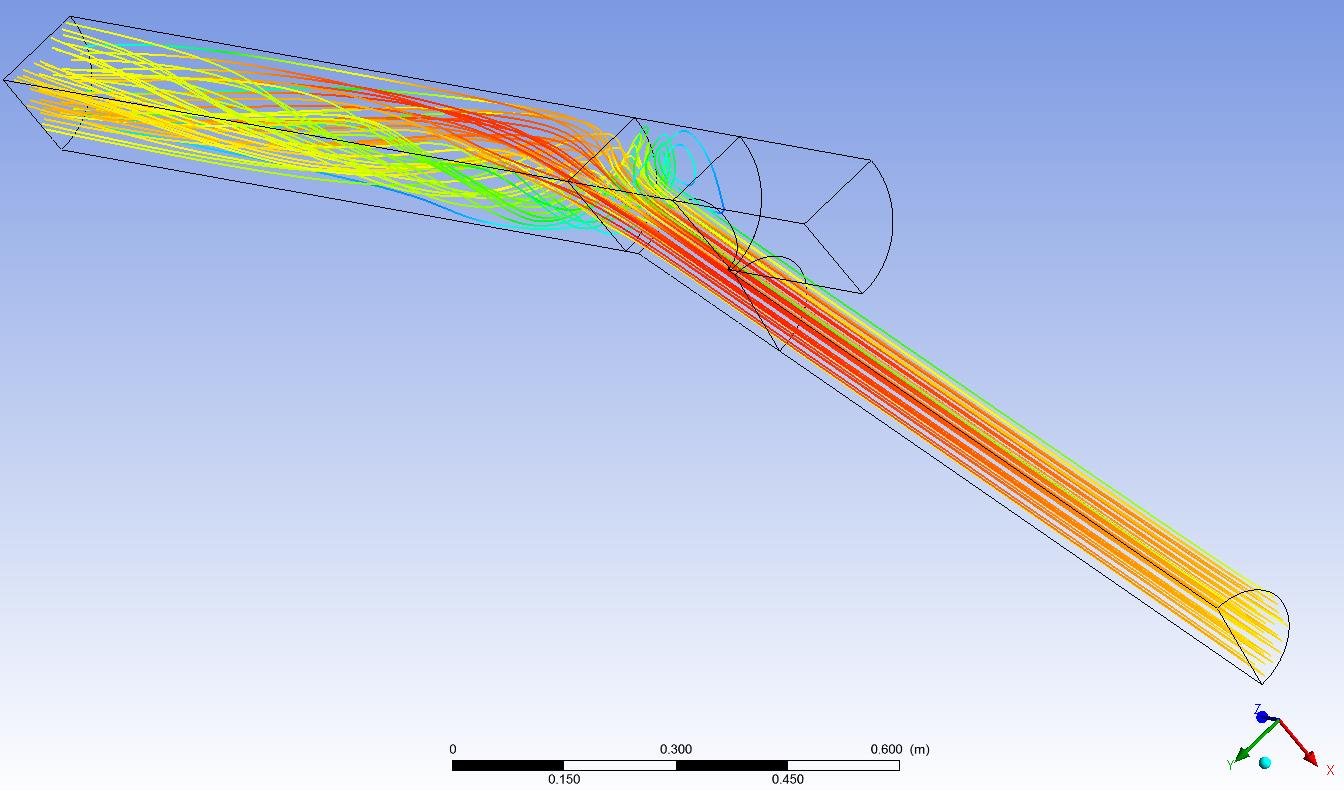Here is the results expanded across the two planes of symmetry.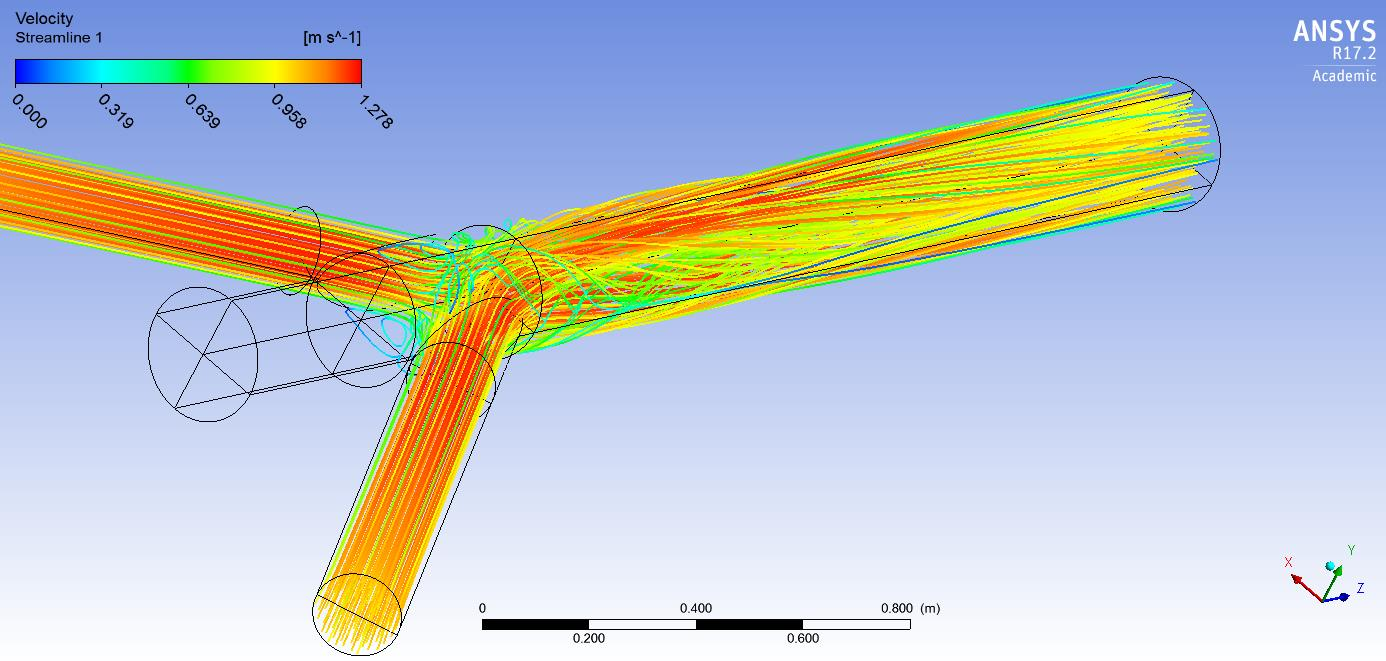• Peter,

Thank you. I do symmetry of a beam, but in results do not show the other side of  beam. And value of displacement is of half beam, i need to divide a displacement with 2 for getting a displacement of whole beam.

You have to check Beta Options in the Workbench Options.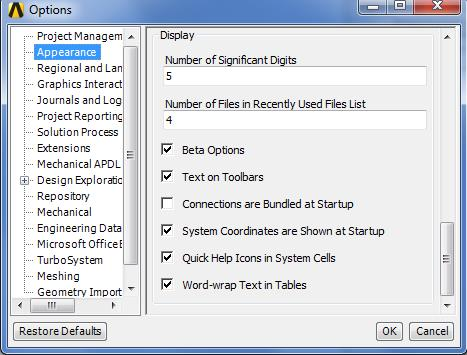Then you get it to work.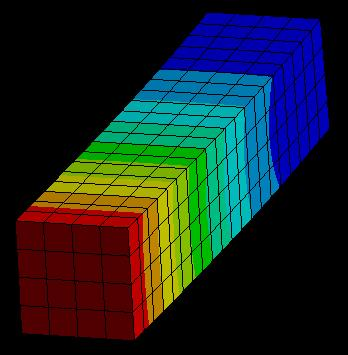When you do a half symmetry for a beam, you put half the load on the beam to get the same end deflection that you would get on a full beam if you applied the full load.

• Peter,

I already included the Beta Options, but I thought that the results will be of whole part.

OK, thank you.

Yes, the results will be the whole part. When I opened your file, there was only 2 elements through the width of the part in the symmetry direction, then I turned on Beta options and now in the results I see 4 elements through the width of the part.

Cheers,
Peter

• edited April 2019

Hello,

Here I have attached a very simple model, where I wanted to check out using symmetry as Peter described (I didn't get how to attach models here in the Forum, so there is a link to a cloud, where I saved it as a wbpz file).

I think, that I have followed Peters description: I created a model and applied two symmetry planes in DM, so now I have a quarter of my original object. I applied a displacement and that worked well. However, I was not able to visualize the full model. Beta Options are turned on, and I put a value of 0.001 at Delta Z (By the way, why do we have to do so? Seems a bit random to me. And always for Delta Z? Or depending on my symmetry plane sometimes also on Delta y or Delta x?)

Then I go to the solution again, but there is still only a quarter of the modell. Has anyone some helpful hints, why I can't visualize the full model?

Regards,

Stephan

•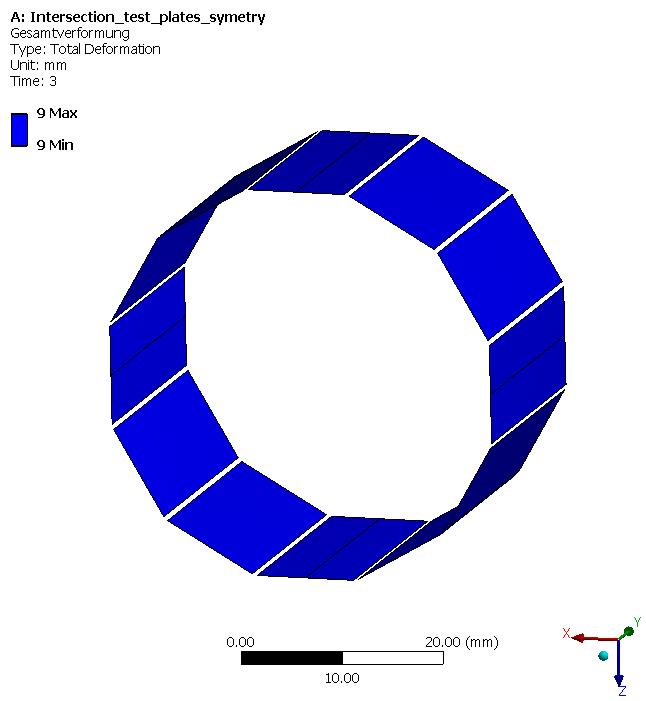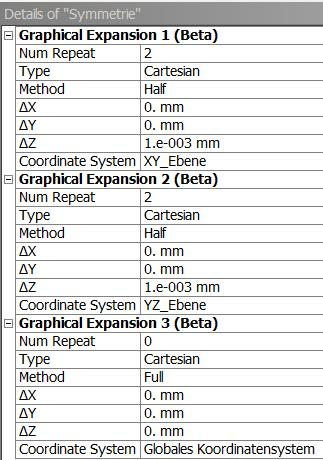• edited April 2019

Thank you! My error was to use the global coordinate System instead of the planes created by the symmetry tool (in my case, the Global XY and YZ- Planes are the geometrically the same as the symmetry planes, so I got a little confused...).

I am still wondering about the 1.e-003 at Delta Z. I mean, I do it, and it works fine, but I don't really know why I'am doing it. Has anyone an explanation for that?

• The need to put in a small delta is a hack for incomplete development of the software. That is why this feature is a Beta option, it's not finished. Maybe the developers will figure out how to make it work with the default 0. Maybe the feature will will move out of the Beta category.

• edited September 2019

Hi Peter

please, I to tried this method but it didn't show me the results as you indicated.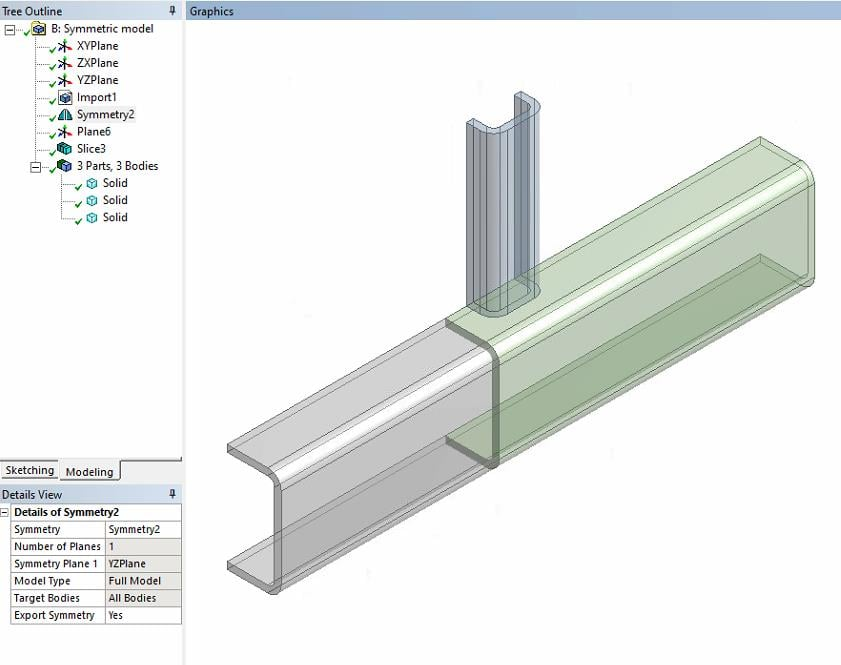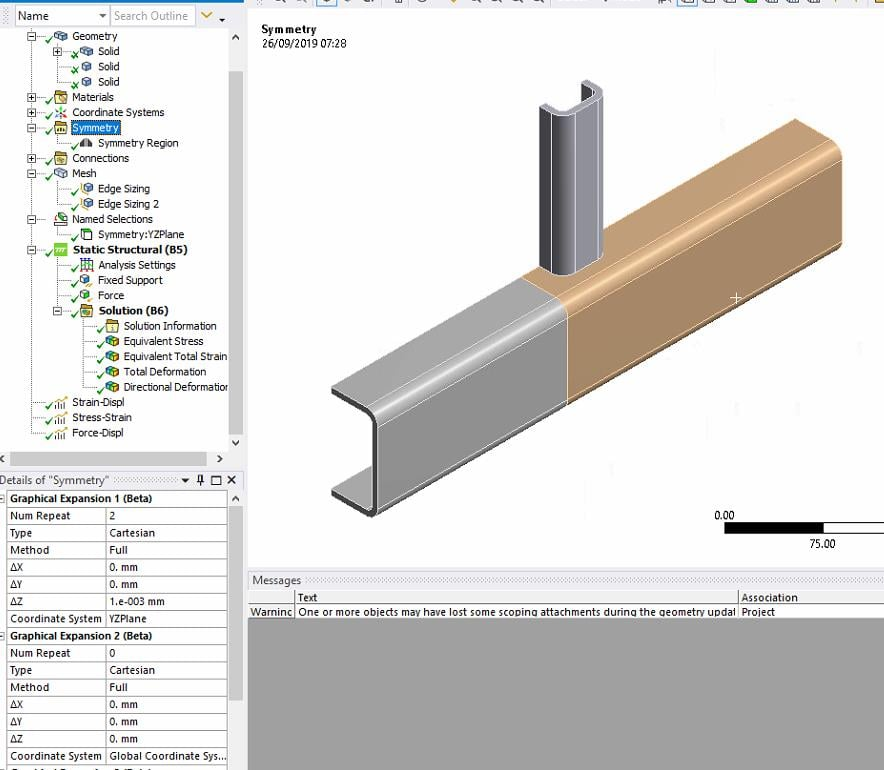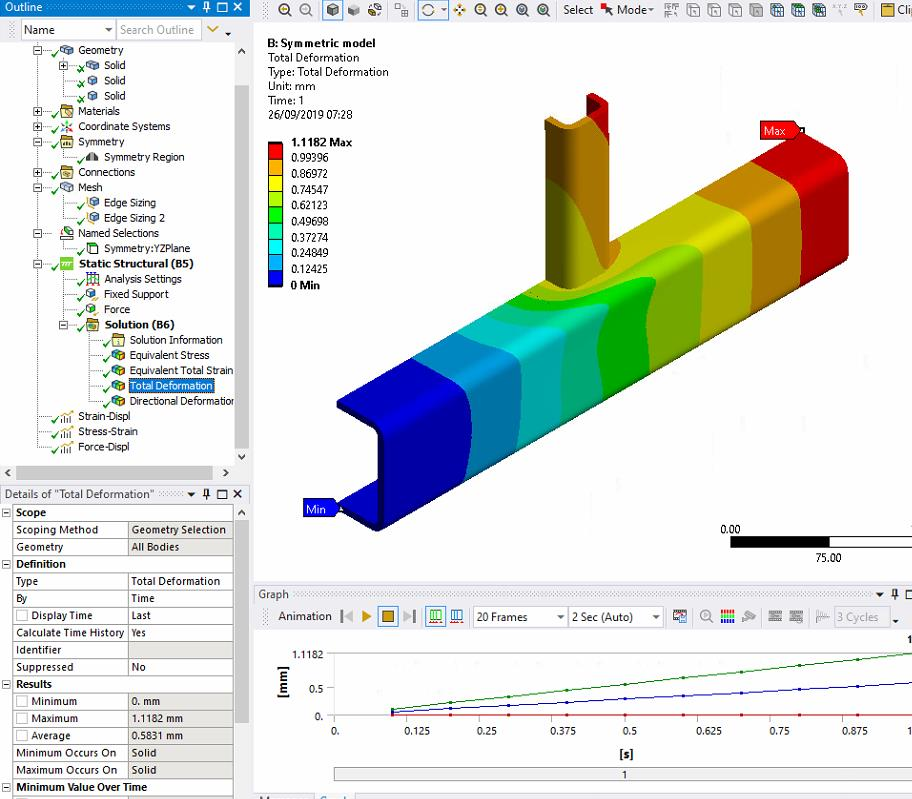• I can't see the Triad in the screen shots above. It looks like the X axis is normal to the plane of symmetry so the 1e-3 should be in the delta X field but maybe it should be -1e03 depending on which way the X axis is pointing. You have 1e-3 in the delta Z field, which is wrong.  You also should change Method from Full to Half.

• edited September 2019

Hi Peter,

I could already obtain the total figure of the model, but the results remain as if it were half a model, to obtain the results as if they were a complete model, I understand that the force and displacement must be multiplied by two, but the stress and strain?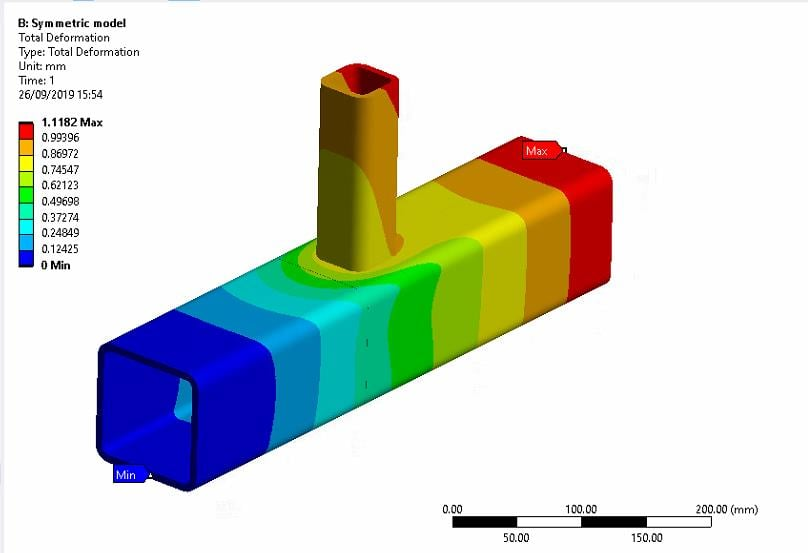• The stress and strain are defined by the deformation.  The symmetry just makes it so the gradient of stress normal to the centerplane is zero.

• edited September 2019

Hi peter

It means that to graph, apart from the deformation, stress and strain should also be multiplied by two (for the previous example that is half of the solid)

Thanks!

• Deformation is local, so is not multiplied by two when 1/2 symmetric model is solved.

Half the force is applied to half the model to get the same local deformation that would occur if the full force was applied to the full model.

That means, when you get reaction force on a half model, you have to multiply it by 2 to get the value for the full model.

• edited November 2019

hello peter

In symmetry Graphical Expansion, why we need Num repeats, shouldn't be always 2 ? Or what is really behind that?

• If you had cyclic symmetry, and modeled a 10 degree slice, then you would need 36 repeats to make a full model.

• edited November 2019

Yeah, I forget to think about that. Thanks, peter• Hello Peter, I have a question regarding symmetry too. I am modeling a reinforced concrete pole, when I applied the simmetry to the full model I noticed reinforcement didn´t dissapear in the simmetry plane, like it is not taken into account. In mechanical, using Ansys 2020 I give the lines the "Reinforcement" property. If I apply simmetry, would the reinforcement be taken into account in the half not shown?

• It would be best to trim each line to the symmetry plane and apply symmetry BCs to the vertices on that plane. For example, if the symmetry plane is XZ and Y is normal, you would apply Displacement Y = 0, Rotation X = 0, and Rotation Z = 0 to every line body vertex on that plane. This is the same for a shell element edge that is cut by the symmetry plane. Solid elements have no rotational DOF at the nodes, so the only BC is Displacement Y = 0.

Once you have symmetry properly defined, the model behaves as if there was a mirror image of the structure on the other side of the plane, enforcing the correct displacements on the half you can see.

In Ansys2020 R2, when you apply Reinforcement property to the line bodies in Mechanical, the nodes on the line bodies automatically connect with the nodes on the adjacent solid elements.